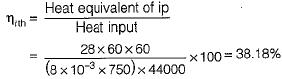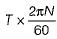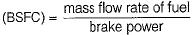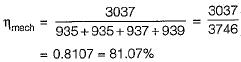Courses

# Test: Testing & Performance - 2

## 10 Questions MCQ Test Topicwise Question Bank for Mechanical Engineering | Test: Testing & Performance - 2

Description
This mock test of Test: Testing & Performance - 2 for Mechanical Engineering helps you for every Mechanical Engineering entrance exam. This contains 10 Multiple Choice Questions for Mechanical Engineering Test: Testing & Performance - 2 (mcq) to study with solutions a complete question bank. The solved questions answers in this Test: Testing & Performance - 2 quiz give you a good mix of easy questions and tough questions. Mechanical Engineering students definitely take this Test: Testing & Performance - 2 exercise for a better result in the exam. You can find other Test: Testing & Performance - 2 extra questions, long questions & short questions for Mechanical Engineering on EduRev as well by searching above.
QUESTION: 1

### A gasoline engine working on Otto cycle consumes 8 litres of gasoline per hour and develops 28 kW. The specific gravity of gasoline. is 0.75 and its calorific value is 44000 kJ/kg. The indicated thermal efficiency of the engine is

Solution:QUESTION: 2

Solution:
QUESTION: 3

### In a variable speed SI engine

Solution:

Since power P =Hence power is directly proportional to speed.

QUESTION: 4

In an SI engine very high compression ratio cannot be used because

Solution:

By increasing the compression ratio, temperature and pressure of the end charge increases. Increase in temperature reduces the delay of the end charge which increases the knocking tendency of SI engine.

QUESTION: 5

The method of determination of indicated power of multi-cylinder SI engine is by the use of

Solution:

Morse test is used for determining indicated power in multi-cylinder engine from which friction power is calculated.

QUESTION: 6

Brake specific fuel consumption is expressed as

Solution:

Brake Specific Fuel ConsumptionIt is generally expressed in kg/kWh

QUESTION: 7

Morse test is applicable only to

Solution:

Morse test is used to determine indicated power for multi-cylinder engine.

QUESTION: 8

The dynamo in an automobile

Solution:
QUESTION: 9

In the context of performance evaluation of IC Engine, match List-I (Parameter) with List-II (Equipment of measurement) and select the correct answer using the codes given below the lists:
List-I
A. Brake power (BHP)
B. Engine speed
C. Calorific value of fuel
D. Exhaust emission
List-II
1. Bomb calorimeter
2. Electrical tachometer
3. Hydraulic dynamometer
4. Flame ionization detector
Codes:
A B C D
(a) 3 1  2 2
(b) 4 2  1 3
(c) 3 2  1 4
(d) 2 3 1  5

Solution:

(i) Brake power → Hydraulic dynamo-meter
(ii) Engine speed → Electrical tachometer
(iii) Calorific value of fuel → Bomb calorimeter
(iv) Exhaust emission → Flame ionization detectors

QUESTION: 10

During a Morse test on a 4-cylinder engine, the following measurements of brake power were taken at constant speed.
All cylinders firing : 3037 kW
Number 1 cylinder not firing : 2102 kW
Number 2 cylinder not firing : 2102 kW
Number 3 cylinder not firing : 2100 kW
Number 4 cylinder not firing : 2098 kW
Q. The mechanical efficiency of the engine is

Solution:

When cylinder 1 is not firing then power is 2102 kW and when all cylinders are firing then power is 3037 kW.
Hence power supplied by cylinder
No. 1 = 3037 - 2102 = 935 kW
Similarly power supplied by cylinder
No. 2 = 3037 - 2102 = 935 kW
Similarly power supplied by cylinder
No. 3 = 3037 - 2100 = 937 kW
Similarly power supplied by cylinder
No. 4 = 3037 - 2098 = 939 kW
Hence